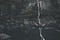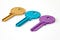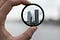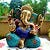# What is Bias-Variance Trade-off in Machine Learning?Image source

In the process of building a Predictive Machine Learning model, we always come across some types of error, either with training data or testing data. Here, let’s understands this error problem in simple words because sometimes, it is difficult to understand the concept with mathematical and technical terms.

# DBMS keysImage source

In the beginning study of the DBMS keys, we are confused about the DBMS keys concepts because there are many different types of DBMS keys, and almost all are related to each other with a slight difference, which makes it very difficult to get a clear understanding of the time. In this blog, you will learn the following concepts.

What are DBMS keys?

Keys in DBMS are an attribute or set of attributes in a database table to uniquely identify a row in the…

# Vanishing and Exploding Gradients in Neural NetworksImage Source

In this blog, you will understand why the Vanishing and Exploding Gradient problem happens. What are Vanishing and Exploding Gradient problems, and why does it occur.

What is a Gradient?

The Gradient is nothing but a derivative of loss function with respect to the weights. It is used to updates the weights to minimize the loss function during the back propagation in neural networks.

What is Vanishing Gradients?

Vanishing Gradient occurs when the derivative or slope will get smaller and smaller as we go backward with every layer during backpropagation.

When weights update is very small or exponential small, the…

# What is a Confusion Matrix in Machine Learning?Image source

A Confusion matrix is an N x N matrix used for evaluating the performance of a classification model, where N is the number of target classes. The matrix compares the actual target values with those predicted by the machine learning model.

For a binary classification, the confusion matrix is 2 x 2 as shown below with 4 output:

# What is Logistic Regression?

Logistic regression is used when the dependent variable is categorical. For example,

Consider a scenario where we need to classify whether the tumor is malignant or benign. If we use linear regression for this problem, there is a need for setting up a threshold based on which classification can be done. Say if the actual class is malignant, predicted continuous value 0.4 and the threshold value is 0.5, the data point will be classified as benign which can lead to…

# What is Random Forest Regression?

Random Forest or Random Decision Forests are an ensemble learning method for classification and regression tasks and other tasks that operate by constructing a multitude of decision trees at training time and outputting the class that is the mode of the classes (classification) or mean prediction (regression) of the individual trees.

Let’s understand Random Forest Regression using the Position_Salaries data set which is available on Kaggle. This data set consists of a list of positions in a company along with the band levels and their associated salary. …

# What is Decision Tree Regression?

Decision trees are a non-parametric supervised learning method used for both classification and regression tasks. Decision trees are constructed via an algorithmic approach that identifies ways to split a data set based on different conditions. It is one of the most widely used and practical methods for supervised learning.

Let’s understand Decision Tree Regression using the Position_Salaries data set which is available on Kaggle. This data set consists of a list of positions in a company along with the band levels and their associated salary. …

# What is Support Vector Regression?

Support Vector Machine is a supervised machine learning algorithm that can be used for regression or classification problems. It can solve linear and non-linear problems and work well for many practical problems. It uses a technique called the kernel trick to transform your data and then based on these transformations it finds an optimal boundary between the possible outputs.

Let’s understand Support Vector Regression using the Position_Salaries data set which is available on Kaggle. This data set consists of a list of positions in a company along with the band levels and their associated salary. …

# What is a Polynomial Linear Regression?

Polynomial Linear Regression is similar to the Multiple Linear Regression but the difference is, in Multiple Linear Regression the variables are different whereas in Polynomial Linear Regression, we have the same variable but it is in a different power.

Why it is called a Linear Regression if it’s a Polynomial Regression?

Polynomial regression fits a nonlinear relationship between the value of x and the corresponding conditional mean of y, denoted E(y | x). In which x is non-linear because it is in a different power but here we talking about the coefficient. …

# Regression Algorithm part2: Multiple Linear Regression Using R Language

What is a Multiple Linear Regression?

Multiple Linear Regression is an extension of the simple linear regression algorithms to predict values from more than one independent variable. So in general it is a relationship between multiple independent variable and one dependent variable.

Let’s understand Multiple Linear Regression using the 50-startups data set which is available on Kaggle. This data set contains 50 business startups data. The variables used in the data set are R&D spend, Administration, and Marketing Spend, State and Profit. Our goal is to design a model that can predict the Profit based on appropriate independent variable.

# Required R package

First…## Margi patel

Data Scientist/Machine learning Engineer, Writer